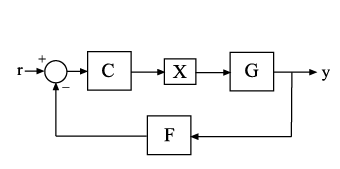# showTunable

Display current value of tunable Control Design Blocks in Generalized Model

## Syntax

``showTunable(M)``

## Description

example

````showTunable(M)` displays the current values of all tunable Control Design Blocks in a generalized LTI model. Tunable control design blocks are parametric blocks such as `realp`, `tunableTF`, and `tunablePID`.```

## Examples

collapse all

Tune the following control system using `systune`, and display the values of the tunable blocks.The control structure includes a PI controller `C` and a tunable low-pass filter in the feedback path. The plant `G` is a third-order system.

Create models of the system components and connect them together to create a tunable closed-loop model of the control system.

```s = tf('s'); num = 33000*(s^2 - 200*s + 90000); den = (s + 12.5)*(s^2 + 25*s + 63000); G = num/den; C0 = tunablePID('C','pi'); a = realp('a',1); F0 = tf(a,[1 a]); X = AnalysisPoint('X'); T0 = feedback(G*X*C0,F0); T0.InputName = 'r'; T0.OutputName = 'y';```

`T0` is a `genss` model that has two tunable blocks, the PI controller, `C`, and the parameter, `a`. `T0` also contains the switch block `X`.

Create a tuning requirement that forces the output `y` to track the input `r`, and tune the system to meet that requirement.

```Req = TuningGoal.Tracking('r','y',0.05); [T,fSoft,~] = systune(T0,Req);```
```Final: Soft = 1.43, Hard = -Inf, Iterations = 59 ```

`systune` finds values for the tunable parameters that optimally meet the tracking requirement. The output `T` is a `genss` model with the same Control Design Blocks as `T0`. The current values of those blocks are the tuned values.

Examine the tuned values of the tunable blocks of the control system.

`showTunable(T)`
```C = 1 Kp + Ki * --- s with Kp = 0.000433, Ki = 0.00525 Name: C Continuous-time PI controller in parallel form. ----------------------------------- a = 67.8 ```

`showTunable` displays the values of the tunable blocks only. If you use `showBlockValue` instead, the display also includes the switch block `X`.

## Input Arguments

collapse all

Input model of which to display tunable block values, specified as a generalized LTI model such as a `genss` model.

## Tips

• Displaying the current values of tunable blocks is useful, for example, after you have tuned the free parameters of the model using a tuning command such as `systune`.

• `showTunable` displays the current values of the tunable blocks only. To display the current values of all Control Design Blocks in a model, including tunable, uncertain, and switch blocks, use `showBlockValue`.